# Multiplication - 7th grade (12y) - math problems

#### Number of problems found: 338

• Gas priceIf a cm3 of gas costs rm 1.50, how many cents would a liter of gas cost? 1 rm = 1 Malaysian Ringgit = 100 Malaysian Ringgit cents = equals 0.21 Euro in 2021/Q3
• Central Park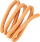Central Park is a rectangular park in New York City and the map scale is 1 cm: 320 m (It means 1 cm on the map represents 320 m in the real world. ). Find the north-south distance if the length on the map is 2.5 cm.
• The space museum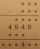The science club is going to the space museum. The club consists of 27 students. If admission costs \$6.50 for children ages 4–11 and \$8.50 for children ages 12–17, how much will it cost for the entire science club's admission to the museum? Science Club S
• Nathan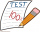Nathan is playing a trivia game. Each correct answer is worth 2 ½ points, and each incorrect answer is worth -¼ points. If he gets 35 questions correct and 32 questions incorrect, how many points does he have? A. 87.5 B. 79.5 C. 75 D. 8
• A giraffe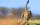A giraffe can run up to 46.93 feet per second. How far could a giraffe run in 1.8 seconds?
• Yellow tangs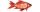In an aquarium 2/5 of the fish are surgeonfish.  Of these 3/4 are yellow tangs.  What fraction of all fish in the aquarium are yellow tangs?
• A land 2A land owner owns two parcels of land measuring 4 2/5 hectares and 6 1/5 hectares. He sells 3/4 of the total land. How many hectares of the land are left?
• The product 3The product of two numbers is 15 ⁵/₆. If one of the numbers is 5 ²/₃, find the other.
• AlexandraAlexandra made a rectangular quilt the measured 3 1/4. 2 3/4 feet in width. What is the area of the quilt in square feet? Write an equation to solve.
• The product 2The product of two functions is 10. If one of them is 2 1/3, find the other one.
• Simplify expression with mixedWhen (7¹/3 + 2¾)÷(2 + 2¼ × 1¹/3) is simplified the result is?
• Evaluate fractionsThe difference  of 5 1/2 and 2/3 is added to the product of 5/6 and 1/2
• English and math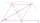1/5 of a class study English, and 3/5 study mathematics. If the class consist of 80 students, how many students study English and mathematics in the class?
• Sign of a expressionWhat is the sign of expression: minus 18 start fraction 9 divided by 17 end fraction plus left parenthesis minus 18 start fraction 9 divided by 17 end fraction right parenthesis?
• A farmer 3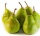A farmer sells 9.3 kg of pears and apples at the farmers market.3/4 of this weight is pears, and the rest is apples. How many kilograms of apples did she sell at the farmers market.
• Product increased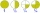When the product of 2/3 and 6/10 is increased by 2/5, the result is?
• Clothes 4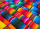If the cost of 16.5 metres of cloth is ₹2,062.50 then find the cost of 24.75 metres of clothes
• Long and wideWhat is the area of a rectangle that is 1 1/4 meters long and 3/4​ of a meter wide
• Four multiplesFour multiples of 6 he writes the following 12  24  56  72, which is correct?
• A number 5A number is divisible by 24, 25, and 120 if it is increased by 20. Find the number.

Do you have an exciting math question or word problem that you can't solve? Ask a question or post a math problem, and we can try to solve it.

We will send a solution to your e-mail address. Solved examples are also published here. Please enter the e-mail correctly and check whether you don't have a full mailbox.

Multiplication Problems. Examples for 7th grade (seventh).